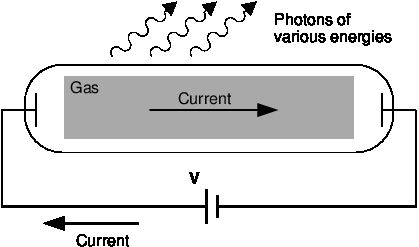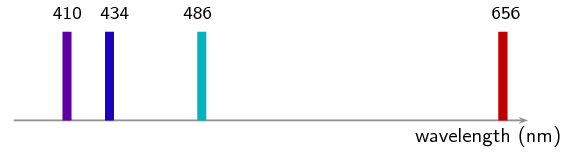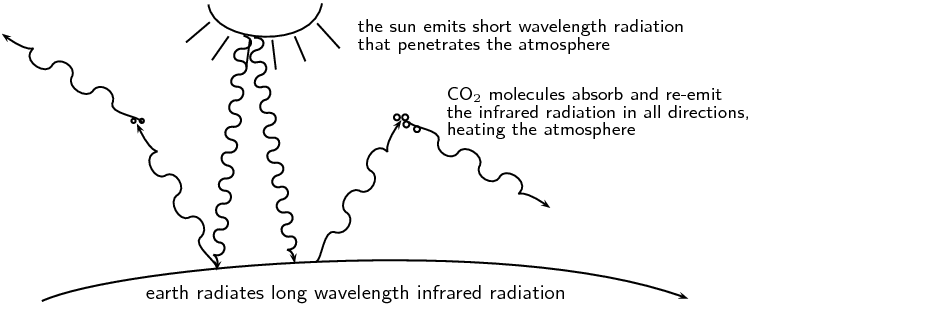We think you are located in United States. Is this correct?

# 12.3 Emission and absorption spectra

## 12.3 Emission and absorption spectra (ESCQR)

### Emission spectra (ESCQS)

You have learnt previously about the structure of an atom. The electrons surrounding the atomic nucleus are arranged in a series of levels of increasing energy. Each element has a unique number of electrons in a unique configuration therefore each element has its own distinct set of energy levels. This arrangement of energy levels serves as the atom's unique fingerprint.

In the early 1900s, scientists found that a liquid or solid heated to high temperatures would give off a broad range of colours of light. However, a gas heated to similar temperatures would emit light only at certain specific wavelengths (colours). The reason for this observation was not understood at the time.

Scientists studied this effect using a discharge tube.Figure 12.5: Diagram of a discharge tube. The tube is filled with a gas. When a high enough voltage is applied across the tube, the gas ionises and acts like a conductor, allowing a current to flow through the circuit. The current excites the atoms of the ionised gas. When the atoms fall back to their ground state, they emit photons to carry off the excess energy.

A discharge tube (shown in Figure 12.5) is a gas-filled, glass tube with a metal plate at both ends. If a large enough voltage difference is applied between the two metal plates, the gas atoms inside the tube will absorb enough energy to make some of their electrons come off, i.e. the gas atoms are ionised. These electrons start moving through the gas and create a current, which raises some electrons in other atoms to higher energy levels. Then as the electrons in the atoms fall back down, they emit electromagnetic radiation (light). The amount of light emitted at different wavelengths, called the emission spectrum, is shown for a discharge tube filled with hydrogen gas in Figure 12.6 below. Only certain wavelengths (i.e. colours) of light are seen, as shown by the lines in the picture.Figure 12.6: Diagram of the emission spectrum of hydrogen in the visible spectrum. Four lines are visible, and are labelled with their wavelengths. The three lines in the $$\text{400}$$–$$\text{500}$$ $$\text{nm}$$ range are in the blue part of the spectrum, while the higher line ($$\text{656}$$ $$\text{nm}$$) is in the red/orange part.

Eventually, scientists realised that these lines come from photons of a specific energy, emitted by electrons making transitions between specific energy levels of the atom. Figure 12.7 shows an example of this happening. When an electron in an atom falls from a higher energy level to a lower energy level, it emits a photon to carry off the extra energy. This photon's energy is equal to the energy difference between the two energy levels ($$\Delta E$$).

$\Delta E_{\text{electron}} = E_f - E_i$

As we previously discussed, the frequency of a photon is related to its energy through the equation $$E=hf$$. Since a specific photon frequency (or wavelength) gives us a specific colour, we can see how each coloured line is associated with a specific transition.Figure 12.7: In the first diagram are shown some of the electron energy levels for the hydrogen atom. The arrows show the electron transitions from higher energy levels to lower energy levels. The energies of the emitted photons are the same as the energy difference between two energy levels. You can think of absorption as the opposite process. The arrows would point upwards and the electrons would jump up to higher levels when they absorb a photon of the right energy. The second representation shows the wavelengths of the light that is emitted for the the various transitions. The transistions are grouped into a series based on the lowest level involved in the transition.

Visible light is not the only kind of electromagnetic radiation emitted. More energetic or less energetic transitions can produce ultraviolet or infrared radiation. However, because each atom has its own distinct set of energy levels (its fingerprint!), each atom has its own distinct emission spectrum.

temp text

### Absorption spectra (ESCQT)

Atoms do not only emit photons; they also absorb photons. If a photon hits an atom and the energy of the photon is the same as the gap between two electron energy levels in the atom, then the electron in the lower energy level can absorb the photon and jump up to the higher energy level. If the photon energy does not correspond to the difference between two energy levels then the photon will not be absorbed (it can still be scattered).

Using this effect, if we have a source of photons of various energies we can obtain the absorption spectra for different materials. To get an absorption spectrum, just shine white light on a sample of the material that you are interested in. White light is made up of all the different wavelengths of visible light put together. In the absorption spectrum there will be gaps. The gaps correspond to energies (wavelengths) for which there is a corresponding difference in energy levels for the particular element.

The absorbed photons show up as black lines because the photons of these wavelengths have been absorbed and do not show up. Because of this, the absorption spectrum is the exact inverse of the emission spectrum. Look at the two figures below. In Figure 12.8 you can see the line emission spectrum of hydrogen. Figure 12.9 shows the absorption spectrum. It is the exact opposite of the emission spectrum! Both emission and absorption techniques can be used to get the same information about the energy levels of an atom.

The dark lines correspond to the frequencies of light that have been absorbed by the gas. As the photons of light are absorbed by electrons, the electrons move into higher energy levels. This is the opposite process of emission.

The dark lines, absorption lines, correspond to the frequencies of the emission spectrum of the same element. The amount of energy absorbed by the electron to move into a higher level is the same as the amount of energy released when returning to the original energy level.

## Worked example 4: Absorption

I have an unknown gas in a glass container. I shine a bright white light through one side of the container and measure the spectrum of transmitted light. I notice that there is a black line (absorption line) in the middle of the visible red band at $$\text{642}$$ $$\text{nm}$$. I have a hunch that the gas might be hydrogen. If I am correct, between which 2 energy levels does this transition occur? (Hint: look at Figure 12.7 and the transitions which are in the visible part of the spectrum.)

### What is given and what needs to be done?

We have an absorption line at $$\text{642}$$ $$\text{nm}$$. This means that the substance in the glass container absorbed photons with a wavelength of 642 nm. We need to calculate which 2 energy levels of hydrogen this transition would correspond to. Therefore we need to know what energy the absorbed photons had.

### Calculate the energy of the absorbed photons

\begin{align*} E& = \frac{hc}{\lambda }\\ & = \frac{\left(\text{6,63} \times \text{10}^{-\text{34}}\right)\times \left(\text{3} \times \text{10}^{\text{8}}\right)}{\text{642} \times \text{10}^{-\text{9}}}\\ & = \text{3,1} \times \text{10}^{-\text{19}}\text{ J} \end{align*}

The absorbed photons had an energy of $$\text{3,1} \times \text{10}^{-\text{19}}$$ $$\text{J}$$.

### Find the energy of the transitions resulting in radiation at visible wavelengths

Figure 12.7 shows various energy level transitions. The transitions related to visible wavelengths are marked as the transitions beginning or ending on Energy Level 2. Let us find the energy of those transitions and compare with the energy of the absorbed photons we have just calculated.

Energy of transition (absorption) from Energy Level 2 to Energy Level 3:

\begin{align*} \Delta E_{electron} = {E}_{2,3}& = {E}_{2}-{E}_{3}\\ & = \text{16,3} \times \text{10}^{-\text{19}}- \text{19,4} \times \text{10}^{-\text{19}}\\ & = -\text{3,1} \times \text{10}^{-\text{19}}\text{ J} \end{align*}

Therefore the energy of the photon that an electron must absorb to jump from Energy Level 2 to Energy Level 3 is $$\text{3,1} \times \text{10}^{-\text{19}}$$ $$\text{J}$$. (NOTE: The minus sign means that absorption is occurring.)

This is the same energy as the photons which were absorbed by the gas in the container! Therefore, since the transitions of all elements are unique, we can say that the gas in the container is hydrogen. The transition is absorption of a photon between Energy Level 2 and Energy Level 3.

The energy of the photon does not correspond to the energy of an energy level, it corresponds to the difference in energy between two energy levels.

temp text

### Applications of emission and absorption spectra (ESCQV)

The study of spectra from stars and galaxies in astronomy is i called spectroscopy. Spectroscopy is a tool widely used in astronomy to learn different things about astronomical objects.

#### Identifying elements in astronomical objects using their spectra

Measuring the spectrum of light from a star can tell astronomers what the star is made of. Since each element emits or absorbs light only at particular wavelengths, astronomers can identify what elements are in the stars from the lines in their spectra. From studying the spectra of many stars we know that there are many different types of stars which contain different elements and in different amounts.

#### Determining velocities of galaxies using spectroscopy

You have already learnt in Chapter 6 about the Doppler effect and how the frequency (and wavelength) of sound waves changes depending on whether the object emitting the sound is moving towards or away from you. The same thing happens to electromagnetic radiation (light). If the object emitting the light is moving towards us, then the wavelength of the light appears shorter (called blueshifted). If the object is moving away from us, then the wavelength of its light appears stretched out (called redshifted).

The Doppler effect affects the spectra of objects in space depending on their motion relative to us on the earth. For example, the light from a distant galaxy that is moving away from us at some velocity will appear redshifted. This means that the emission and absorption lines in the galaxy's spectrum will be shifted to a longer wavelength (lower frequency). Knowing where each line in the spectrum would normally be if the galaxy was not moving and comparing it to the redshifted position, allows astronomers to precisely measure the velocity of the galaxy relative to the Earth.

#### Global warming and greenhouse gases

The sun emits radiation (light) over a range of wavelengths that are mainly in the visible part of the spectrum. Radiation at these wavelengths passes through the gases of the atmosphere to warm the land and the oceans below. The warm earth then radiates this heat at longer infrared wavelengths. Carbon dioxide (one of the main greenhouse gases) in the atmosphere has energy levels that correspond to the infrared wavelengths that allow it to absorb the infrared radiation. It then also emits at infrared wavelengths in all directions. This effect stops a large amount of the infrared radiation from getting out of the atmosphere, which causes the atmosphere and the earth to heat up. More radiation is coming in than is getting back out.So increasing the amount of greenhouse gases in the atmosphere increases the amount of trapped infrared radiation and therefore the overall temperature of the earth. The earth is a very sensitive and complicated system upon which life depends and changing the delicate balances of temperature and atmospheric gas content may have disastrous consequences if we are not careful.

## Emission and absorption spectra

Textbook Exercise 12.2

Explain how atomic emission spectra arise and how they relate to each element on the periodic table.

Atomic emission spectra arise from electrons dropping from higher energy levels to lower energy levels within the atom, photons (light packets) with specific wavelengths are released. The energy levels in an atom are specific/unique to each element on the periodic table therefore the wavelength of light emitted can be used to determine which element the light came from.

How do the lines on the atomic spectrum relate to electron transitions between energy levels?

The lines on the atomic spectrum relate to electron transitions between energy levels, if the electron drops an energy level a photon is released resulting in an emission line and if the electron absorbs a photon and rises an energy level an absorption line is observed on the spectrum.

Explain the difference between atomic absorption and emission spectra.

The difference between absorption and emission spectra are that absorption lines are where light has been absorbed by the atom thus you see a dip in the spectrum whereas emission spectra have spikes in the spectra due to atoms releasing photons at those wavelengths.

Describe how the absorption and emission spectra of the gases in the atmosphere give rise to the Greenhouse Effect.

The following needs to be in your answer: in what wavelength range the sunlight reaches the earth, the absorption of the sunlight and the re-radiation as infrared light, and finally the scattering of the infrared light by the carbon-dioxide and how this scattering contributes to the Greenhouse Effect.

What colour is the light emitted by hydrogen when an electron makes the transition from energy level 5 down to energy level 2? (Use Figure 12.7 to find the energy of the released photon.)

\begin{align*} \Delta E & = E_5 - E_2 \\ & = \text{21,0} \times \text{10}^{-\text{19}}\text{ J} - \text{16,3} \times \text{10}^{-\text{19}}\text{ J} \\ & = \text{4,7} \times \text{10}^{-\text{19}}\text{ J} \end{align*}

\begin{align*} \lambda & = \frac{hc}{\Delta E} \\ & = \frac{(\text{6,63} \times \text{10}^{-\text{34}}\text{ m$^{2}$kg·s$^{-1}$})(\text{3} \times \text{10}^{\text{8}}\text{ m·s$^{-1}$})}{\text{4,7} \times \text{10}^{-\text{19}}\text{ J}} \\ & = \text{423}\text{ nm} \end{align*} The colour of light emitted at $$\text{423}$$ $$\text{nm}$$ is violet.

I have a glass tube filled with hydrogen gas. I shine white light onto the tube. The spectrum I then measure has an absorption line at a wavelength of 474 nm. Between which two energy levels did the transition occur? (Use Figure 12.7 in solving the problem.)

\begin{align*} \Delta E & = \frac{hc}{\lambda} \\ & = \frac{(\text{6,63} \times \text{10}^{-\text{34}}\text{ m$^{2}$kg·s$^{-1}$})(\text{3} \times \text{10}^{\text{8}}\text{ m·s$^{-1}$})}{\text{474} \times \text{10}^{-\text{9}}\text{ nm}}\\ & = \text{4,20} \times \text{10}^{-\text{19}}\text{ J} \end{align*} This energy interval corresponds to a transition from energy level 4 to energy level 2.